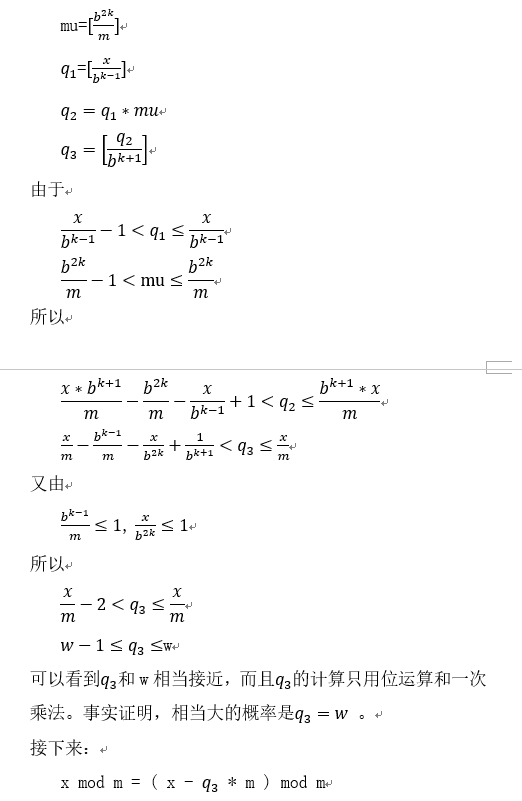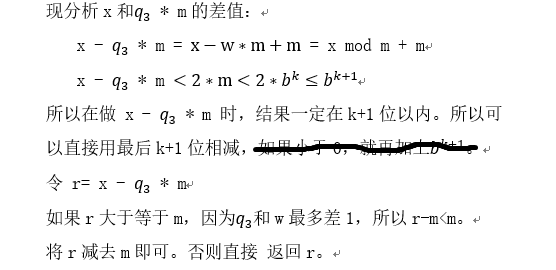# 大数取模运算，快速幂取模运算

### 1.快速幂取模

http://www.cnblogs.com/yinger/archive/2011/06/08/2075043.html

long exp_mod(long a,long n,long b)
{
long t;
if(n==0) return 1%b;
if(n==1) return a%b;
t=exp_mod(a,n/2,b);
t=t*t%b;
if((n&1)==1) t=t*a%b;
return t;
}

### 2.大数取模运算Barrett reduction

https://blog.csdn.net/ykry35/article/details/79179285

Barrett reduction算法的证明和使用。

m的位数是k

q1 = x / b^(k-1)

q2 = q1 * mu

q3 = q2 / b^(k+1)

r1 = x % b^(k+1)

r2 = (q3 * m) % b^(k+1)

r = r1 - r2

if ( r > m ) r -= m

return r

[]表示取整，b是大数的进制### 大数取模：一般取模+技巧取模+快速幂取模+欧拉函数(费马小定理)

https://blog.csdn.net/u011361880/article/details/77802742

(a^n)%m。 我们可以改写为(a^n)%m= (（a%m)^n)%m, 即循环n次。

int exp_mod(int a,int n,int m){
a = a%m;
int temp = 1;
while(n--)
{
temp = temp * a;
temp = temp % m;
}
return temp;
}

(a^n)%10

a%10. 有10种可能，（来源于室友“张博士”的逆天发现，明明可以靠脸吃饭，他偏偏要靠才华，明明可以快速幂取模，它偏要发现这种，出现大大大大数的）

a%10 = 0. 这个结果就不需要看了。0
a%10 = 1. (1^n )%10会出现的可能数字：1
a%10 = 2. (2^n )%10会出现的可能数字：2，4（=2*2）， 8（=2*4），6（=2*8），继续循环，2, 4, 8, 6…..

a%10 = 3. (3^n )%10会出现的可能数字：3，9（=3*3），7（=3*9）， 1（=3*7）,继续循环，3,9,7,1…..

a%10 = 4. (4^n )%10会出现的可能数字：4，6（4*4） , 继续循环，4,6……

a%10 = 5. (5^n )%10会出现的可能数字：5
a%10 = 6. (6^n )%10会出现的可能数字：6
a%10 = 7. (7^n )%10会出现的可能数字：7, 9, 3, 1 继续循环，
a%10 = 8. (8^n )%10会出现的可能数字：8, 4, 2, 6 继续循环，
a%10 = 9. (9^n )%10会出现的可能数字：9, 1 继续循环，

OK

(a^n)%10 = (a^（n%4+4）)%10 = ((a%10)^（n%4+4）) % 10

http://www.cnblogs.com/yinger/archive/2011/06/08/2075043.html

(a^n) %b

long exp_mod(long a,long n,long b)
{
long t;
if(n==0) return 1%b;
if(n==1) return a%b;
t=exp_mod(a,n/2,b);
t=t*t%b;
if((n&1)==1) t=t*a%b;
return t;
}

http://www.cnblogs.com/yinger/archive/2011/06/08/2075043.html

(a^n) %b

int exp_mod(int a,int b,int n){
int r=1;
while(b){
if(b&1)r=(r*a)%n;
a=(a*a)%n;
b>>=1;       // b = b>>1;
}
return r;
}

OK，假设b == 16. a==2.
b = 16,8,4,2,1

b=16，进入while a = 2^2;
b=8, a = 2^4；
b=4, a = 2^8;
b=2, a = 2^16
b=1, ，进入if语句，r = a, 此时返回的是r。

b=18，进入while a = 2^2;
b=9, 此时，进入了if语句。r=2^2. a = 2^4；
b=4, a = 2^8;
b=2, a = 2^16
b=1, ，进入if语句，r = r*a = 2^2 * 2^16 , 此时返回的是r。

r保存的刚好是这个值。

b=22 进入while a = 2^2
b=11 进入if，r = 2^2. a=2^4 ——>要补回2^2
b=5 进入if， r=2^2 * 2^4. a = 2^8 ——> 要补回2^4
b=2 a=2^16
b=1,进入if， r = 2^2 * 2^4 * 2^16

（10到11，在b=11那里已经补回来了），再到22，翻倍，变成2个。

φ(8)=4，因为1,3,5,7均和8互质。

(a^n)%1000000007 = (a^（ k * 1000000006 +r ）)%1000000007
=(a^（ k * 1000000006 ）)%1000000007 * (a^r )%1000000007
=1 * (a^r )%1000000007

04-268822
04-301万+04-052599
07-311万+
11-011万+
07-20891
08-282万+
07-281万+
05-14423
05-281111